# The Snowplow Problem

One morning it begins snowing heavily, and continues snowing at a constant rate. At noon a snowplow starts down a long road, clearing the snow as it goes. At 1:00 a second plow starts at the same place and follows the first plow, clearing the snow that has fallen since the first plow passed. At 2:00 the second plow catches up to the first. When did it start snowing?It sounds like we don't have the information to answer this question. In fact, we don't: some assumptions must be made. We assume:

1.
there was no snow on the road before the time t0 when it started snowing.
2.
there is no snow on the road immediately after a plow passes.
3.
the plow can clear a constant amount of snow per unit time.

Thus a plow's speed is inversely proportional to the depth of snow, which is proportional to the time since t0 (for the first plow) or the time since the first plow passed (for the second plow).

For the first plow, the differential equation is thuswhere k is constant, and x is distance down the road. Actually it's best to think of t as a function of x rather than vice versa: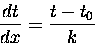with initial condition (measuring time in hours from noon) t(0) = 0. The solution isCall this t1. Now the differential equation for the second plow, again expressing t as a function of x, is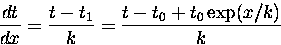with initial condition t(0) = 1. This is a linear equation, and its solution is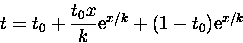Call this t2. The two plows meet where t1 = t2, i.e.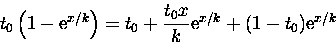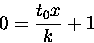x = -k/t0

Plugging in this value for x, we have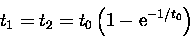This, the time when the plows meet, is supposed to be 2. The equation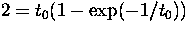can't be solved in closed form'', but numerical solution is possible (e.g. by Newton's method). The result is t0 = -.7959050950, i.e. it started snowing -.7959050950 hours before noon, or about 11:12 am.

Note: the assumption that the plow clears a constant amount of snow per unit time can't really be true when the depth of the snow is small. In fact, according to the differential equation the second plow is moving at infinite speed when it meets the first plow! If this problem has anything to do with reality, we hope that the equation works until the two plows are very close to each other. Then the inaccuracy due to the equation not working in the last few seconds before the plows meet (as the second snowplow tries to accelerate like an Indy car) may not affect the final result too badly.